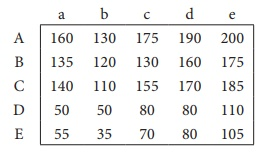Home | | Business Maths 12th Std | Miscellaneous Problems

# Miscellaneous Problems

Book back answers and solution for Exercise questions - Maths : Operations Research: Miscellaneous Problems with Answer, Solution

Miscellaneous Problems

1. The following table summarizes the supply, demand and cost information for four factors S1, S2, S3, S4. shipping goods to three warehouses D1,D2, D3Find an initial solution by using north west corner rule. What is the total cost for this solution?

2. Consider the following transportation problemDetermine an initial basic feasible solution using (a) Least cost method (b) Vogelâ€™s approximation method.

3. Determine an initial basic feasible solution to the following transportation problem by using (i)North West Corner rule (ii) least cost method.4. Explain Vogelâ€™s approximation method by obtaining initial basic feasible solution of the following transportation problem.5. A car hire company has one car at each of five depots a,b,c,d and e. A customer in each of the fine towers A,B,C,D and E requires a car. The distance (in miles) between the depots (origins) and the towers(destinations) where the customers are given in the following distance matrix.How should the cars be assigned to the customers so as to minimize the distance travelled?

6. A natural truck-rental service has a surplus of one truck in each of the cities 1,2,3,4,5 and 6 and a deficit of one truck in each of the cities 7,8,9,10,11 and 12. The distance(in kilometers) between the cities with a surplus and the cities with a deficit are displayed below:How should the truck be dispersed so as to minimize the total distance travelled?

7. A person wants to invest in one of three alternative investment plans: Stock, Bonds and Debentures. It is assumed that the person wishes to invest all of the funds in a plan. The pay-off matrix based on three potential economic conditions is given in the following table:Determine the best investment plan using each of following criteria i) Maxmin ii) Minimax.